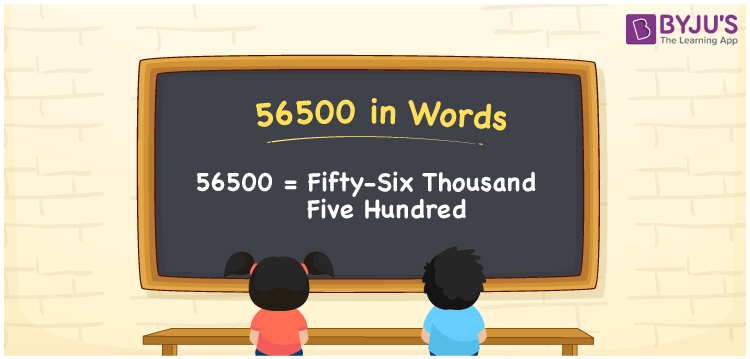# 56500 in words

56500 in words is written as Fifty Six Thousand Five Hundred . In 56500, 5 has a place value of ten thousand, 6 is in the place value of thousand, 5 is in the place value of hundred. The article on Place Value gives more information. The number 56500 is used in expressions that relate to money, distance, length, social media views, and many more. For example, “The serial number on a ticket read the number as Fifty Six Thousand Five Hundred.” Another example, “An MBA intern had to distribute Fifty Six Thousand Five Hundred pamphlets as part of a project.”

 56500 in words Fifty Six Thousand Five Hundred Fifty Six Thousand Five Hundred in Numbers 56500

## 56500 in English Words## How to Write 56500 in Words?

We can convert 56500 to words using a place value chart. The number 56500 has 5 digits, so let’s make a chart that shows the place value up to 5 digits.

 Ten thousand Thousands Hundreds Tens Ones 5 6 5 0 0

Thus, we can write the expanded form as:

5 × Ten thousand + 6 × Thousand + 5 × Hundred + 0 × Ten + 0 × One

= 5 × 10000 + 6 × 1000 + 5 × 100 + 0 × 10 + 0 × 1

= 56500.

= Fifty Six Thousand Five Hundred.

56500 is the natural number that is succeeded by 56499 and preceded by 56501.

56500 in words – Fifty Six Thousand Five Hundred.

Is 56500 an odd number? – No.

Is 56500 an even number? – Yes.

Is 56500 a perfect square number? – No.

Is 56500 a perfect cube number? – No.

Is 56500 a prime number? – No.

Is 56500 a composite number? – Yes.

## Solved Example

1. Write the number 56500 in expanded form

Solution: 5 x 10000 + 6 x 1000 + 5 x 100 + 0 x 10 + 0 x 1

Or Just 5 x 10000 + 6 x 1000 + 5 x 100

We can write 56500 = 50000 + 6000 + 500 + 0 + 0

= 5 x 10000 + 6 x 1000 + 5 x 100 + 0 x 10 + 0 x 1.

## Frequently Asked Questions on 56500 in words

Q1

### How to write the number 56500 in words?

56500 in words is written as Fifty Six Thousand Five Hundred.
Q2

### Is 56500 divisible by 3?

No. 56500 is not divisible by 3.
Q3

### Is 56500 divisible by 10?

Yes. 56500 is divisible by 10.